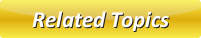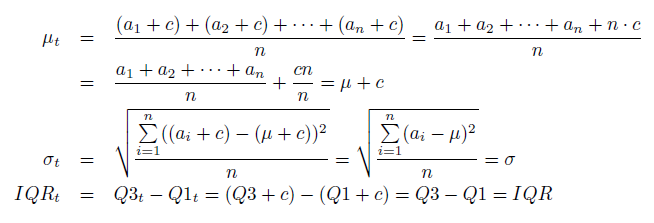# Linear Transformations

When every element undergoes multiplication or increased by a constant it is called as linear transformation. Linear transformation impacts standard deviation, mean, IQR and other crucial numerals in numerous ways.

## Sum of Linear Transformations

Whenever a constant c are summed up to each extremity (member) of a set the mean would be c more than it was prior to the constant was summed. The variance and standard deviation won't be impacted and the IQR won't be impacted. We'll prove this facts now, having μ and σ be the standard deviation & mean, on the other hand, prior to adding c and μt and σt be the standard deviation & mean and after the shift. Lastly, we allow the original set be {a1, a2, . . . , an}, hence the translated set is {a1 + c, a2 + c, . . . , an + c}.Here we apply the solution of the 1st equation to substitute μt with μ+c in the 2nd equation. As the variance is merely the standard deviation's square, the reality is the standard deviation is not impacted implies that the variance will not be either.

## Linear Transformation Multiplication

A different type of shift is multiplication. If every member of a set by multiplication process by a constant c, and then the mean would be c times its value prior to the constant was multiplied, the standard deviation would be |c| times its value prior to constant undergoes multiplication and IQR would be |c| times its value. By applying the same notation like before, we get:Latest Articles

Average Acceleration Calculator

Average acceleration is the object's change in speed for a specific given time period. ...

Free Fall Calculator

When an object falls into the ground due to planet's own gravitational force is known a...

Permutation

In Mathematics, the permutation can be explained as the arrangement of objects in a particular order. It is an ordered...

Perimeter of Rectangle

A rectangle can be explained as a 4-sided quadrilateral which contains equal opposite sides. In a rectangle

Perimeter of Triangle

A three sided polygon which has three vertices and three angles is called a triangle. Equilateral triangle...